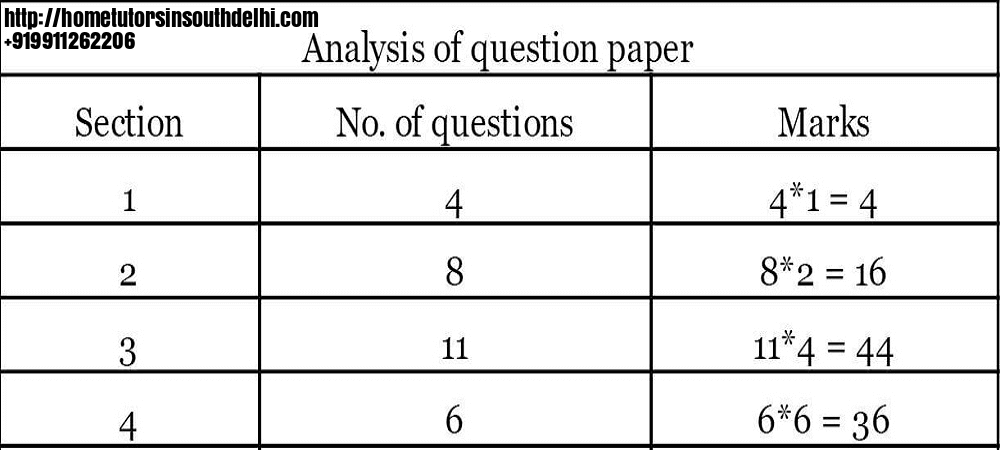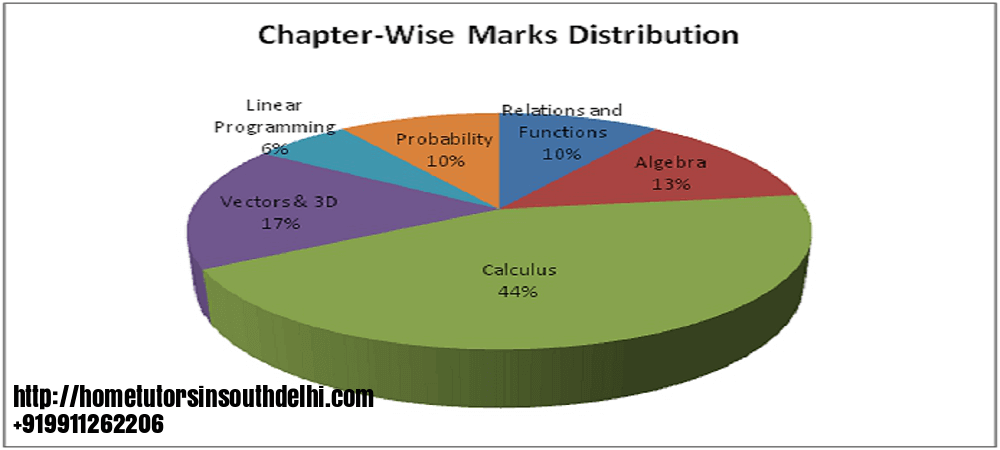# Mathematics Home Tutors

Maths Home Tutors play an important role in nurturing the academic life of a student. Mathematics is probably the most popular and also a very important subject in class 11-12.

## Maths Home Tutors

Maths is an integral part of Science stream non-medical group. Science medical group needs to study physical maths to understand their Physics and Chemistry properly. Mathematics is also very important for commerce group students because Delhi University requires Mathematics at +2 level for doing B.Com(H). And Mathematics also plays a very crucial role in competitive exams. Also, we need a lot of practice in Mathematics so that the student can cover all the varieties of problems posed in the exam paper. Even Humanities students are studying Mathematics at +2 level.### Mathematics Home Tutors help and support students by following ways:-

►Maths home tutors advise students to make a proper study plan for the mathematics board exam. with a strong and well-defined planning,  students can score maximum marks and they can finish their paper within the given time.

►Our tutors advise that students should mention the correct property when solving questions of calculus and algebra. definite integration and the inverse of a matrix are the areas which require a lot of practice. this work can be easily done with the help of our maths home tutors.

►Students can clear their concepts thoroughly with our tutor to secure full marks in vectors and 3d. And all formulas of 3d should be written on a separate sheet practiced again and again.►Above image and table shows the syllabus break-up and paper pattern. mathematics home tutors help students get prepared accordingly

►Our tutors focus on using advanced technology in their teaching. We are posting this video as an example.

►The way you present your work is really important to score full in probability. mathematics home tutors make students practice many extra questions on conditional probability